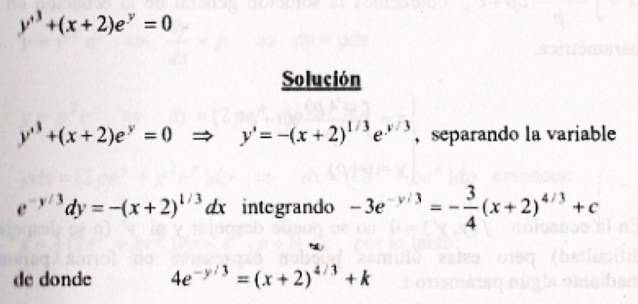Is this the right solution for the ODE

Homework Statement

well the problem is to solve de following differential equation.

##y'^3+(x+2)e^y=0##

##y'=dy/dx=p##

The Attempt at a Solution

I got this problem in my test today, an i did it just like it is in the image below, but my teacher wasn't sure that it was a correct way of solving it, i would like to know if it is, and if it's not and how to solve it them.I got this from a book of solutions of de T.Mackarenko, and I think is right but i know that the constant in the end when you have integrated has to show the highest power of ##p## in this case 3, but also i don't know if in this case it changes because i made it a different equation in which ##p## was to the power of 1. Thank you

Last edited:

HallsofIvy
Homework Helper
I don't know what you mean by " the constant in the end when you have integrated has to show the highest power of p in this case 3". The constant of integration is just that- a constant- a number. It doesn't "show a power"

I don't know what you mean by " the constant in the end when you have integrated has to show the highest power of p in this case 3". The constant of integration is just that- a constant- a number. It doesn't "show a power"

Well i've learned that the constant of integration at the end of a differential equation is going to show you the power to which the derivative was. Is like in algebraic equations when you factorize a polynomial of the 5th power when you take the factorization back out you will get again a polynomial of the 5th power. The same i was toughed with differential equations, if your ODE power is 3, you will end up with a constant to the power of 3. Sorry if my english is not clear.

But, is the solution correct?

Redbelly98
Staff Emeritus